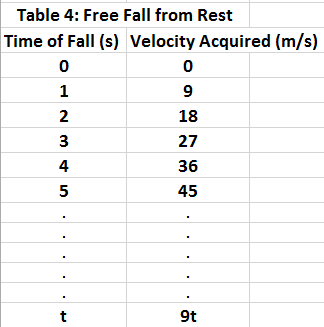# Gravity and Free-Falling Bodies

### Gravity and Free-Falling Bodies

In the absence of friction, all bodies, large or small, heavy or light,
fall to earth at the same rate with the same acceleration and is called acceleration due to gravity.
Such bodies are in a state of free fall.

Acceleration due to Gravity (g) = 9.80 m/s2
The instantaneous speed (or velocity) v of an object falling from rest after a time t is v = gt
The equation indicates that the speed increases by 9.8 m/s in each second of fall.
The table below shows how the speed (or velocity) changes each second. In here, we rounded off g to 10 m/s2.### Example

A ball is dropped from a roof of the house which takes 5 seconds to hit the ground.
Question: Calculate the velocity before the ball crashes to the ground. (g=10m/s2)
Solution:
Assume gravity g = 10m/s2
Velocity is: V=g × t = 10m/s2 × 5s=50m/s.